# Parameter identification of oscillations in power systems based on improved variational modal decomposition and HTLS-adaline method

## Chunlu Wan1, Kang Wang2, Zhixiang Wu3

1Artificial Intelligence School, Wuchang University of Technology, Wuhan, 430223, China

2College of Information Engineering, Wuchang Institute of Technology, Wuhan, 430065, China

3College of Computer Science and Technology, Wuhan University of Science and Technology, Wuhan, 430065, China

1Corresponding author

Journal of Vibroengineering, Vol. 24, Issue 1, 2022, p. 143-157. https://doi.org/10.21595/jve.2021.21871
Received 12 January 2021; received in revised form 13 April 2021; accepted 24 April 2021; published 5 November 2021

Copyright © 2021 Chunlu Wan, et al. This is an open access article distributed under the Creative Commons Attribution License, which permits unrestricted use, distribution, and reproduction in any medium, provided the original work is properly cited.
Views 72
WoS Core Citations 0
CrossRef Citations 0
Abstract.

Oscillation has become one of the important problems faced by modern power grids. Multi-types of oscillations may occur simultaneously in the power system and the oscillation frequency span is extremely large. For signals with wide-band oscillation modes, the signals in different frequency bands are first separated by a band-pass filter, and then the Improved Variational Mode Decomposition (IVMD) method with high noise robustness is used to extract each oscillating mode signal. Finally, the combinations of Hankel total least squares (HTLS) and adaptive neural network algorithm (Adaline ANN) is used to estimate the frequency, attenuation factor, amplitude and phase of low-frequency oscillations. Furthermore, the introduction of Adaline neural network solves the problem that the mode amplitude and phase are difficult to determine after IVMD processing, so that the detection accuracy is improved. Simulation and case analysis show that this method can effectively distinguish and extract different types of oscillation modes in the signal, and accurately identify the information of each mode. The IVMD-HTLS-Adaline method can effectively identify signals that have experienced severe oscillations or noise-like signals with potential oscillations.

Keywords: variational mode decomposition, power system, parameter identification, Adaline, oscillation.

#### 1. Introduction

With the large-scale access of renewable energy, the widespread application of power electronic equipment, and the large-scale interconnection of AC and DC in modern power grids, the resource allocation is optimized and the system reliability is improved; the weak links in the system increase and the anti-interference performance decreases. In recent years, new types of faults have continued to emerge, and security and stability issues have become increasingly prominent [1-3]. Oscillation is one of the issues that threatens the stable operation of the power system. Typical oscillations in current power systems include Low-frequency oscillations (LFO) [4-6] caused by regional or interval weak damping and sub-synchronous Oscillation (SSO) caused by series compensation capacitors or energy interaction between the power electronics equipment and generator set [7-10]. In addition, Super-synchronous Oscillation (SurSO) occasionally occurs with sub-synchronous oscillation . Normally, oscillation can be tested in four ways: mechanism, acoustics, electrics and electromagnetic [12-19]. Here, we will focus on the electrical signal waves.

According to different damping, the oscillation signals of power system can be divided into two categories: (1) Maintain or diverge the oscillation signal (when the system is weakly damped or negatively damped); (2) Damped oscillation signal (when the system is in positive damping). The former occurs less frequently, but once it occurs, it will cause great harm to the system. The latter occurs more frequently and is easily masked by noise, also known as noise-like oscillation signals. The rapid and real-time extraction of modal information from the oscillating signal is helpful to the adjustment of power grid dispatching and control strategies. Before the oscillation, the mode identification can be found from the noise-like oscillation signal, and the potential oscillation mode of the system can also be found to provide an early warning for the system. Oscillation mode identification is the key of real-time efficient control and risk early warning of power systems.

Frequency, damping ratio, oscillation amplitude and phase are the key information of oscillation mode, as well as the key parameters to realize oscillation monitoring, early warning, control and protection. At present, the research methods of power system oscillation mainly include model analysis and measurement analysis. The model analysis method depends on the precise model of the system. Large-scale systems and non-linear power electronic devices will affect the accuracy of the model, leading to analysis errors . Measurement analysis analyzes the actual measurement data of the system, and the results are close to the operating conditions. Combining measured data with signal processing technology is a common method for power system oscillation identification.

The Prony algorithm can directly extract the characteristic quantity of the signal to identify more accurate oscillation modal information [21, 22], but it is highly sensitive to noise. In actual engineering, the filtering algorithm is often combined with the Prony algorithm, and the dimensionality of the signal is reduced through the filtering algorithm, or the modal signal is extracted from it. Mean filtering (Average Filter, AF), empirical mode decomposition, autoregressive moving average algorithm, wavelet method and singular value decomposition are commonly used filtering algorithms, which have been applied to the identification of oscillation modes [23-29]. However, the mean filtering can only reduce the noise interference and cannot remove the noise. The essence of wavelet denoising is to fit the signal in a specific frequency band. If there are multiple oscillation modes with similar frequencies, the wavelet method cannot distinguish them. Methods such as Hilbert transform and singular value decomposition may produce large errors when processing low signal-to-noise ratio signals. In addition, in the face of multi-types of coexisting pan-band oscillations with large oscillation frequency spans, the above methods often identify high amplitude oscillations during processing, and other oscillations may be considered as noise and ignored. How to carry out unified modal identification of pan-band oscillation signals and extract modal information of multiple types of oscillations is an important issue for modal identification of power systems.

Variational Mode Decomposition (VMD) algorithm can effectively separate modal signals and is not sensitive to noise . Based on the Improved VMD (Improved VMD, IVMD) method, a method for identifying pan-band oscillations in complex power systems is proposed. First, different types of band-pass filters are used for separation. Secondly, the modal signals in each band-pass filtered signal are extracted using IVMD. Finally, the Hankel total least squares (HTLS) algorithm and the adaptive artificial neural network (Adaline Artificial Neural Network, Adaline ANN) are used to estimate the frequency, attenuation factor, amplitude, and phase of low-frequency oscillations respectively, so as to achieve unified identification of pan-band oscillations. Meanwhile, the performance of the proposed algorithm is verified by simulation and measurement examples.

#### 2. Modal identification framework for Broad-Band oscillation

The oscillation may include multiple oscillation modal signals in different frequency bands and noise signals generated by measurement or the system itself. The original measurement signal with broad-band oscillation can be expressed as ${y}_{0}\left(t\right)$:

(1)
${y}_{0}\left(t\right)=\sum _{n=1}^{N}{y}_{n}\left(t\right)+{y}_{Noise}\left(t\right).$

In Eq. (1): ${y}_{Noise}\left(t\right)$ is the noisy signal, and is the modal signals for $N$ different frequency bands, and:

(2)
${y}_{n}\left(t\right)={A}_{n}\left(t\right){e}^{-{D}_{n}{f}_{n}t}\mathrm{c}\mathrm{o}\mathrm{s}\left(2\pi {f}_{n}t+{\theta }_{n}\right).$

In Eq. (2): ${A}_{n}\left(t\right)$ is the amplitude of the $n$th oscillation mode at time $t$; ${\theta }_{n}$ is the initial phase; ${D}_{n}$ and ${f}_{n}$ are the damping ratio and frequency of oscillation respectively.

#### 2.2. Modal identification framework

The framework for modal oscillations identification in a broad-band is shown in Fig. 1. The identification process is divided into the following three steps.

Step-1. Multiple Band Pass Filters (BPF) is used to decompose the original signal into multiple filtered signals in different frequency bands, so as to separate the oscillation signals.

Step-2. The IVMD algorithm is used to extract the oscillation modal signal from each BPF filtered signal.

Step-3. The HTLS-Adaline algorithm is used to identify the oscillation mode signals provided by IVMD, and obtain the information of each oscillation mode. The original signal is filtered through band-pass filtering, IVMD modal signal extraction and HTLS-Adaline identification to obtain modal parameters.

Fig. 1. Framework of the mode identification for broad-band oscillation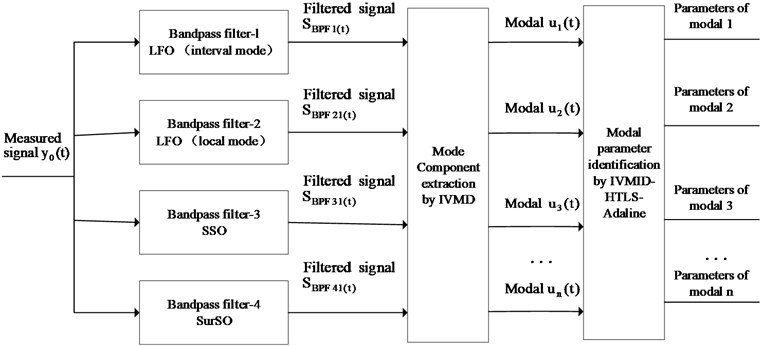#### 3.1. Band-pass filter design

There are mainly three types of oscillations that cause power systems accidents: low-frequency oscillation, sub-synchronous oscillation and super-synchronous oscillation. The low-frequency oscillation is further divided into local mode and interval mode. The frequencies of each type of oscillations are in different ranges. Thus, this article designs four BPFs. A proper signal length selection can improve the accuracy of pattern recognition and ensure the rapidity of recognition. Because each oscillation frequency is different, it is obviously inappropriate to use the same length of data for identification. Table 1 shows the BPF filter bandwidth and identification sampling time corresponding to various oscillations in this paper.

Table 1. Time lengths and frequency bands for different BPFs

 Band-pass filter Oscillation type Sampling time / s Oscillation frequency / Hz BPF-1 LFO (interval modal) 10 0.2-1 BPF-2 LFO (local mode) 2 1-5 BPF-3 SSO 0.4 10-50 BPF-4 SurSO 0.4 70-110

According to the analysis of the modal identification algorithm , if it is necessary to obtain the oscillation frequency and amplitude more accurately, the sampling time of the identification signal must be greater than 1 oscillation period. In order to obtain accurate damping ratio information, the sampling time needs to be longer than two oscillation periods. Therefore, for low-frequency oscillations, in order to ensure rapid identification, the minimum band-pass frequency of the band-pass filter is 0.2 Hz, and the sampling time is selected to be twice the oscillation period, that is 10 s. For sub-synchronous oscillation, the identification speed is considered comprehensively. The minimum oscillation frequency is 10 Hz as the benchmark and the sampling time is 4 times. The oscillation period is 0.4 s. The mechanism of super-synchronous oscillation determines that it appears in pairs with sub-synchronous oscillation and the frequencies are complementary. The sampling time of the synchronous oscillation is the same as that of the sub-synchronous oscillation, and is also 0.4 s.

#### 3.2.1. VMD algorithm

The VMD algorithm is a new adaptive signal decomposition method proposed by Dragomiretskiy et al. in 2014. The target modal is solved by the inherent modal function . In view of the accuracy and noise robustness of the VMD algorithm, this paper uses the VMD method to extract and separate modal signals. The variational problem corresponding to the VMD algorithm is to find the KIMF components with the smallest sum of the estimated bandwidth. The variational problem is transformed into an augmented Lagrange equation and the equation is solved by the Alternating Direction Method of Multipliers (ADMM) to obtain the solution of the modal function ${u}_{k}^{n+1}\left(t\right)$:

(3)
${\stackrel{^}{u}}_{k}^{n+1}\left(\omega \right)=\left(\stackrel{^}{f}\left(\omega \right)-\sum _{i\ne k}{\stackrel{^}{u}}_{i}\left(\omega \right)+\frac{\stackrel{^}{\lambda }\left(\omega \right)}{2}\right)×\frac{1}{1+2\alpha \left(\omega -{\omega }_{k}{\right)}^{2}}.$

Similarly, the solution of the center frequency value of the modal component is:

(4)
${\omega }_{k}^{n+1}=\frac{{\int }_{0}^{\infty }\omega |{\stackrel{^}{u}}_{k}\left(\omega \right){|}^{2}d\omega }{{\int }_{0}^{\infty }|{\stackrel{^}{u}}_{k}\left(\omega \right){|}^{2}d\omega },$

where, $\left\{{\omega }_{k}\right\}=\left\{{\omega }_{1},{\omega }_{2},\cdots ,{\omega }_{K}\right\}$ and $\left\{{\omega }_{k}\right\}=\left\{{\omega }_{1},{\omega }_{2},\cdots ,{\omega }_{K}\right\}$ is the component and its center frequency respectively. The flow of VMD algorithm is as follows:

Step 1. Initialize $\left\{{\omega }_{k}\right\}=\left\{{\omega }_{1},{\omega }_{2},\cdots ,{\omega }_{K}\right\}$ and $n=0$.

Step 2. $n←n+1$, update ${u}_{k}$ and ${\omega }_{k}$ according to Eq. (3) and Eq. (4).

Step 3. Update $\lambda$:

(5)
${\stackrel{^}{\lambda }}^{n+1}\left(\omega \right)←{\stackrel{^}{\lambda }}^{n}\left(\omega \right)+\tau \left(\stackrel{^}{f}\left(\omega \right)-\sum _{k}{\stackrel{^}{u}}_{k}^{n+1}\left(\omega \right)\right).$

Step 4. Repeat steps 2 and 3 until the iteration stop condition is satisfied with $\sum _{k}{‖{\stackrel{^}{u}}_{k}^{n+1}-{\stackrel{^}{u}}_{k}^{n}‖}_{2}^{2}/{‖{\stackrel{^}{u}}_{k}^{n}‖}_{2}^{2}<\epsilon$. End the loop and output the results to get $K$ modal components and their center frequencies.

#### 3.2.2. Determination of VMD penalty factor based on PSO algorithm optimization

The penalty factor $\alpha$ in the VMD algorithm has a great impact on the decomposition results. The study found that the smaller the penalty parameter $\alpha$, the larger the bandwidth of each IMF (Intrinsic Mode Function) component, and vice versa . Therefore, when using VMD to decompose the oscillation signal of the power system, it is very important to choose the appropriate penalty factor parameter $\alpha$. In this paper, genetic mutation particle swarm optimization is used to optimize the penalty parameters to obtain the optimal $\alpha$.

Particle swarm optimization is a global optimization algorithm proposed by Eberh and Kennedy et al. in 1995. This method is a swarm intelligent optimization algorithm, and it has the advantages of few parameters, easy adjustment, and easy to fall into a local optimum. In order to obtain the global optimal approximate solution , this paper introduces the idea of genetic algorithm mutation in particle swarm algorithm to construct a genetic mutation particle swarm algorithm.

Definition of genetic mutation particle swarm algorithm: In an $D$-dimensional search space, label population $X$, and it is composed of m particles, $\mathbf{X}=\left[{\mathbf{x}}_{1},{\mathbf{x}}_{2},\cdots ,{\mathbf{x}}_{m}\right]$. The position of each particle in the search space can be represented by a $D$-dimensional vector, that is, ${\mathbf{x}}_{i}=\left[{x}_{i1},{x}_{i2},\cdots ,{x}_{iD}\right]$. $D$ is the number of parameters to be optimized, and the moving speed of $i$-th particle is ${\mathbf{v}}_{i}=\left[{v}_{i1},{v}_{i2},\cdots ,{v}_{iD}\right]$. The local extremum of particles is ${\mathbf{p}}_{i}=\left[{p}_{i1},{p}_{i2},\cdots ,{p}_{iD}\right]$; the global extremum of the population is ${\mathbf{G}}_{1}=\left[{g}_{1},{g}_{2},\cdots ,{g}_{D}\right]$, and the sub-global optimal value is ${\mathbf{G}}_{2}=\left[{g\text{'}}_{1},{g\text{'}}_{2},\cdots ,{g\text{'}}_{D}\right]$. The maximum individual optimal algebra is $\mathrm{m}\mathrm{a}\mathrm{x}Age$, and the mutation probability is$q$. In order to prevent particles from falling into the local optimum, it is necessary to record the individual optimal maintaining algebra of the particles during the iteration process. When the individual optimal maintaining algebra does not reach $\mathrm{m}\mathrm{a}\mathrm{x}Age$, each particle updates the position of the next generation by individual local extreme value and global extreme value. The formula is updated as:

(6)
${\mathbf{v}}_{i}^{n+1}=\omega {\mathbf{v}}_{i}^{n}+{c}_{1}\eta \left({\mathbf{p}}_{i}-{\mathbf{x}}_{i}^{n}\right)+{c}_{2}\eta \left({\mathbf{G}}_{1}-{\mathbf{x}}_{i}^{n}\right),$
(7)
${\mathbf{x}}_{i}^{n+1}={\mathbf{x}}_{i}^{n}+{\mathbf{v}}_{i}^{n+1}.$

In the formula, $\omega$ is the inertia weight; $\eta$ is a random number between [0, 1]; ${c}_{1}$ and ${c}_{2}$ are the learning factors respectively that represent the local search ability and the global search ability; $n$ is the number of iterations, and ${\mathbf{v}}_{i}$, ${\mathbf{p}}_{i}$, ${\mathbf{G}}_{1}$ and ${\mathbf{x}}_{i}$ are $D$-dimensional vectors respectively. The determination of the inertia weight $\omega$ of the current number of iterations adopts the linear decreasing weight method proposed by Shi , and the formula is as follows:

(8)
$\omega ={\omega }_{\mathrm{m}\mathrm{a}\mathrm{x}}-\frac{\left({\omega }_{\mathrm{m}\mathrm{a}\mathrm{x}}-{\omega }_{\mathrm{m}\mathrm{i}\mathrm{n}}\right)n}{{n}_{\mathrm{m}\mathrm{a}\mathrm{x}}}.$

In the formula, ${\omega }_{\mathrm{m}\mathrm{a}\mathrm{x}}$ and ${\omega }_{\mathrm{m}\mathrm{i}\mathrm{n}}$ are the maximum and minimum inertia weights; $n$ is the current number of iterations, and ${n}_{\mathrm{m}\mathrm{a}\mathrm{x}}$ is the defined maximum number of iterations. When the individual optimal retention algebra reaches $\mathrm{m}\mathrm{a}\mathrm{x}Age$, the genetic mutation operation is used to update the position and velocity of the particle to make it jump out of the local optimal. Selection of fitness function for genetic mutation particle swarm optimization algorithm: In the parameter optimization, the evaluation criterion of the decomposition effect of VMD method uses the concept of envelope entropy ${E}_{p}$ proposed by Tang Gui-ji et al. . The envelope entropy of $x\left(j\right)$ time signal $c$ of length $N$ is defined as:

(9)

In the formula, $a\left(j\right)$ is the envelope signal of $x\left(j\right)$ after Hilbert demodulation, and $j=1,2,\cdots ,N$. ${p}_{j}$ is the result of normalizing $a\left(j\right)$. Normalization not only avoids the influence of different envelope amplitudes of IMF components, but also reduces the interference of weak noise. ${E}_{p}$ is obtained according to the information entropy calculation rules. This article measures the decomposition effect of VMD according to ${E}_{p}$.

The VMD method is used to decompose the BPF filtered oscillation signal. When the component contains more noise, the sparseness of the component signal is weak and the envelope entropy is large. On the contrary, when a regular oscillation signal appears in the component, the signal will have strong sparseness, and the calculated envelope entropy is small at this time. Therefore, under the influence of parameter $\alpha$, the minimum entropy ${E}_{p}$ of the $K$ components is selected as the local minimum entropy $\mathrm{m}\mathrm{i}\mathrm{n}{E}_{p}$. The component corresponding to the minimum entropy value contains rich feature information. The local minimum entropy is used as part of the fitness function of the entire search process to find the parameter ${\alpha }_{0}$ corresponding to the global optimal component. Through the above analysis of parameter $\alpha$, a proper $\alpha$ will reduce the iterations of VMD, that is, the VMD method has a high decomposition efficiency. Therefore, it is necessary to achieve the highest decomposition efficiency in the case of the best decomposition effect. This article builds the fitness function based on $\mathrm{m}\mathrm{i}\mathrm{n}{E}_{p}$, add $time$ (iterations) as follows $\mathrm{m}\mathrm{i}\mathrm{n}F=\mathrm{m}\mathrm{i}\mathrm{n}{E}_{p}+\beta \cdot time$, where $\beta$ is the quantization factor of the fitness function.

In this paper, the number of modal components $K$ and penalty factor $A$ in VMD decomposition are set as model hyper parameters. In the process of VMD optimization by PSO, the number of particle is set as 30, and the maximum number of iterations is set as 500.The learning factor is set as 2; the velocity inertia factor is set as $w=$ 0.8, and the velocity coefficient is setas $\lambda =$ 1. The maximum and minimum inertia weights are set as ${\omega }_{\mathrm{m}\mathrm{a}\mathrm{x}}=$ 0.9 and ${\omega }_{\mathrm{m}\mathrm{i}\mathrm{n}}=$ 0.1 respectively, and the quantization factor of the fitness function is set as 1/1000. The parameter optimization process based on genetic mutation particle swarm optimization algorithm is shown in Fig. 2.

Fig. 2. Parameter optimization process based on genetic mulation particle swarm optimization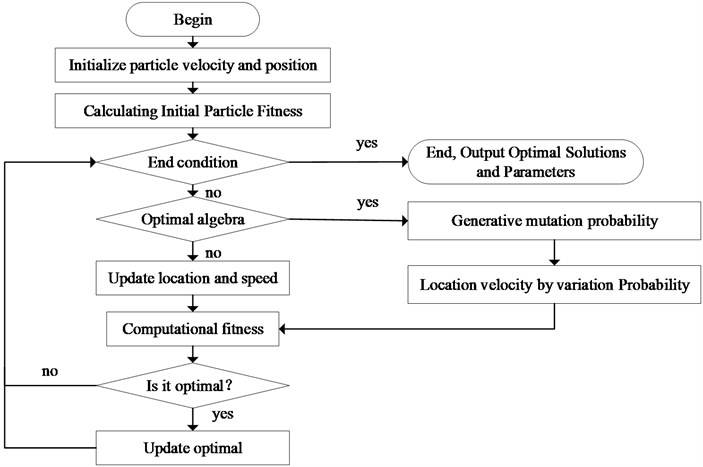The BPF signal is decomposed by improved VMD to obtain the oscillation mode ${u}_{n}\left(t\right)$ extracted by VMD. When the accuracy of BPF and VMD is high enough, for modal signal ${u}_{n}\left(t\right)$, there is ${u}_{n}\left(t\right)\approx {y}_{n}\left(t\right)$.

Therefore, through BPF and IVMD, all oscillation modal signals can be separated from the original signal. The convergence process of VMD optimization using PSO is shown in Fig. 3.

Fig. 3. Convergence process of fitness function in VMD optimization by PSO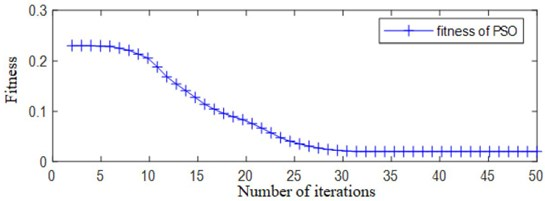#### 3.3. VMD-HTLS algorithm for frequency and attenuation factor

After the modal signals ${u}_{n}\left(t\right)$ are obtained by the IVMD decomposition, the HTLS algorithm is used to identify the modal parameters, such as the oscillation frequency and attenuation factor. HTLS algorithm is a subspace rotation invariant method, which has high computing efficiency and strong anti-noise ability. Its calculation steps are described in . The main idea is to construct a Hankel matrix using the sampled signal, and perform Vander Mang decomposition on it. Using the translation-invariant characteristic of the van der Mun matrix to construct the equivalent relationship, and the eigenvalues of the oscillating modes are obtained. The main idea of IVMD-HTLS is: IVMD is used to decompose the BPF filtered sequence, and then the HTLS algorithm is used to calculate the oscillation frequency and attenuation factor for each component after decomposition. Because the FOMC-HTLS algorithm cannot give the amplitude and phase of the original signal $y\left(n\right)$, and the information of each mode is incomplete. It is not conducive to the reconstruction of the signal and the quantitative evaluation of the algorithm. Therefore, this paper introduces the Adaline God network to oscillate modal information (Amplitude and Phase).

#### 3.4.1. Adaptive linear neural network

Adaptive linear (Adaline) neural network is a neuron model proposed originally by Widrow and Hoff . It is widely used in signal processing and other fields.

Fig. 4. The principle of adaptive linear neural network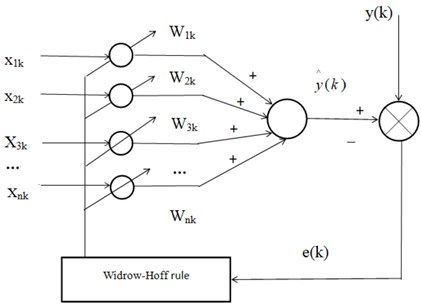In Fig. 4, ${x}_{1k}$, ${x}_{2k}$,⋯, ${x}_{nk}$ are the $n$ input signals of the adaptive linear neural network at time$k$.The input signal vector form is expressed as ${\mathbf{X}}_{ik}=\left[{x}_{1k},{x}_{2k},\cdots ,{x}_{nk}{\right]}^{T}$, and this is often called the input pattern vector of Adaline neural network. The weight vector corresponding to each group of input signals is ${\mathbf{W}}_{ik}=\left[{w}_{1k},{w}_{2k},\cdots ,{w}_{nk}{\right]}^{T}$. The Adaline neural network output is:

(10)
$\stackrel{^}{y}\left(k\right)={\mathbf{X}}_{ik}^{T}{\mathbf{W}}_{ik}.$

Let $A$ be the ideal response signal, and define the error function as:

(11)
$e\left(k\right)=y\left(k\right)-\stackrel{^}{y}\left(k\right).$

The working process of Adaline neural network is as follows : the ideal response signal $y\left(k\right)$ is compared with the output signal $\stackrel{^}{y}\left(k\right)$ of the neural network to obtain different $e\left(k\right)$. Feed$e\left(k\right)$into the learning rules, and adjust the weight vector according to the learning rules to make $\stackrel{^}{y}\left(k\right)$ and $y\left(k\right)$ consistent.

The learning rule of Adaline neural network is Widrow-Hoff rule, which is the least square error algorithm (LMS). The rule weight vector adjustment expression is:

${\mathbf{W}}_{i\left(k+1\right)}={\mathbf{W}}_{i\left(k+1\right)}+\eta e\left(k\right){\mathbf{X}}_{i\left(k+1\right)}.$

In the formula, $\eta$ is the learning rate of the Adaline neural network, $\eta \in \left(0,1\right)$. Its value directly affects the weight vector adjustment accuracy and the convergence velocity.

#### 3.4.2. Solution of oscillation modes by Adaline neural network

The specific steps of Adaline neural network to solve the amplitude and phase are as follows, and a known low-frequency oscillation discrete sampling signal model is established:

(12)

When the attenuation factor and frequency are known, Eq. (12) can be written as:

(13)
$x\left(n\right)=\sum _{i=1}^{M}\left[{A}_{i}{e}^{{\alpha }_{i}n\mathrm{\Delta }t}\mathrm{c}\mathrm{o}\mathrm{s}\left(2\pi {f}_{i}n\mathrm{\Delta }t\right)\mathrm{c}\mathrm{o}\mathrm{s}{\theta }_{i}-{A}_{i}{e}^{{\alpha }_{i}n\mathrm{\Delta }t}\mathrm{s}\mathrm{i}\mathrm{n}\left(2\pi {f}_{i}n\mathrm{\Delta }t\right)\mathrm{s}\mathrm{i}\mathrm{n}{\theta }_{i}\right]$

In the formula, ${p}_{i}={A}_{i}\mathrm{c}\mathrm{o}\mathrm{s}{\theta }_{i}$ and ${q}_{i}={A}_{i}\mathrm{s}\mathrm{i}\mathrm{n}{\theta }_{i}$. The matrix expression of Eq. (13) is:

(14)
$\mathbf{x}=\mathbf{p}\mathbf{C}-\mathbf{q}\mathbf{S}.$

Among them:

$\mathbf{C}=\left[\begin{array}{cccc}\mathrm{c}\mathrm{o}\mathrm{s}\left(2\pi {f}_{1}\mathrm{\Delta }t\right)& \mathrm{c}\mathrm{o}\mathrm{s}\left(2\pi {f}_{1}2\mathrm{\Delta }t\right)& \cdots & \mathrm{c}\mathrm{o}\mathrm{s}\left(2\pi {f}_{1}N\mathrm{\Delta }t\right)\\ \mathrm{c}\mathrm{o}\mathrm{s}\left(2\pi {f}_{2}\mathrm{\Delta }t\right)& \mathrm{c}\mathrm{o}\mathrm{s}\left(2\pi {f}_{2}2\mathrm{\Delta }t\right)& \cdots & \mathrm{c}\mathrm{o}\mathrm{s}\left(2\pi {f}_{2}N\mathrm{\Delta }t\right)\\ ⋮& ⋮& ⋮& ⋮\\ \mathrm{c}\mathrm{o}\mathrm{s}\left(2\pi {f}_{Q}\mathrm{\Delta }t\right)& \mathrm{c}\mathrm{o}\mathrm{s}\left(2\pi {f}_{Q}2\mathrm{\Delta }t\right)& \cdots & \mathrm{c}\mathrm{o}\mathrm{s}\left(2\pi {f}_{Q}N\mathrm{\Delta }t\right)\end{array}\right],$
$\mathbf{S}=\left[\begin{array}{cccc}\mathrm{s}\mathrm{i}\mathrm{n}\left(2\pi {f}_{1}\mathrm{\Delta }t\right)& \mathrm{s}\mathrm{i}\mathrm{n}\left(2\pi {f}_{1}2\mathrm{\Delta }t\right)& \cdots & \mathrm{s}\mathrm{i}\mathrm{n}\left(2\pi {f}_{1}N\mathrm{\Delta }t\right)\\ \mathrm{s}\mathrm{i}\mathrm{n}\left(2\pi {f}_{2}\mathrm{\Delta }t\right)& \mathrm{s}\mathrm{i}\mathrm{n}\left(2\pi {f}_{2}2\mathrm{\Delta }t\right)& \cdots & \mathrm{s}\mathrm{i}\mathrm{n}\left(2\pi {f}_{2}N\mathrm{\Delta }t\right)\\ ⋮& ⋮& ⋮& ⋮\\ \mathrm{s}\mathrm{i}\mathrm{n}\left(2\pi {f}_{Q}\mathrm{\Delta }t\right)& \mathrm{s}\mathrm{i}\mathrm{n}\left(2\pi {f}_{Q}2\mathrm{\Delta }t\right)& \cdots & \mathrm{s}\mathrm{i}\mathrm{n}\left(2\pi {f}_{Q}N\mathrm{\Delta }t\right)\end{array}\right].$

Similarly, define the error function:

(15)
$e\left(n\right)=x\left(n\right)-\stackrel{^}{x}\left(n\right).$

In the formula, $x\left(n\right)$ is the actual sample, and $\stackrel{^}{x}\left(n\right)$ is the output of the neural network. Define the performance indicators as:

(16)
$J=\frac{1}{2}\sum _{n=0}^{N-1}{e}^{2}\left(n\right).$

Because the attenuation factor and frequency are known, $\mathbf{p}$ and $\mathbf{q}$ are unknown in Eq. (14), $\mathbf{C}$ and $\mathbf{S}$ are the input vectors of the neural network. According to the training principle of the steepest descent method, the weight vectors p and q are adjusted to:

(17)
${\mathbf{p}}_{n+1}={\mathbf{p}}_{n}-\eta \frac{\partial J}{\partial {\mathbf{p}}_{n}}={\mathbf{p}}_{n}+\eta {e}_{k}{\mathbf{C}}^{T},$
(18)
${\mathbf{q}}_{n+1}={\mathbf{q}}_{n}-\eta \frac{\partial J}{\partial {\mathbf{q}}_{n}}={\mathbf{q}}_{n}+\eta {e}_{k}{\mathbf{S}}^{T}.$

When the Adaline neural network training is completed, the amplitude and phase of the oscillation mode are solved from the obtained weight vector and Eq. (19):

(19)
$\left\{\begin{array}{l}{A}_{i}=\sqrt{{p}_{n}^{2}\left(i\right)+{q}_{n}^{2}\left(i\right)},\\ {\theta }_{i}=\mathrm{a}\mathrm{r}\mathrm{c}\mathrm{t}\mathrm{a}\mathrm{n}\frac{{q}_{n}\left(i\right)}{{p}_{n}\left(i\right)}.\end{array}\right\$

In this paper, the number of neurons in Adaline neural network is equal to the number $K$ of modal components after VMD decomposition. The activation function of neurons is a constant function, that is, $f=$ 1. The learning rule is the minimum mean square error (LMS) criterion, and the learning rate $\eta =$ 0.0015. The maximum number of network iteration is 5000, and the network iteration stops when the error $\delta$ satisfies $\delta <$ 0.0001.

#### 4.1. Simulation analysis

In order to verify the effectiveness of the method in this paper, an oscillating signal ${y}_{test}$ is constructed by simulation:

${y}_{test}={y}_{LFO1}+{y}_{LFO2}+{y}_{SSO1}+{y}_{SSO2}+{y}_{SurSO}+{y}_{Noise}.$

In the formula: ${y}_{LFO1}$ is the low-frequency oscillation interval mode;${y}_{LFO2}$ is the low-frequency oscillation local mode; ${y}_{SSO1}$ is the sub-synchronous oscillation and paired with the super-synchronous oscillation signal ${y}_{SurSO}$; ${y}_{SSO2}$ is another independent sub-synchronous oscillation, and ${y}_{Noise}$ is the white noise. In line with the real situation, the test signal satisfies the following conditions and assumptions: (1) All oscillation frequencies are randomly selected within the frequency band of the oscillation type; (2) Low frequency oscillation is the main mode of oscillation; the amplitude is higher than the sub-synchronous oscillation, and the local mode of low frequency oscillation is higher than the interval mode; (3) In the pair of sub-synchronous and super-synchronous oscillations, the amplitude of sub-synchronous oscillation mode is slightly higher than the super-synchronous mode. Based on the above assumptions, the specific parameters of each mode of the test signal are finally selected as:

That is, the test signal ${y}_{test}$ contains 5 oscillating signals with different frequencies and a white noise signal with amplitude of 0.16. The frequency band of the test signal oscillation ${y}_{test}$ is 0.63-93.42 Hz. Each test signal is equal amplitude oscillation. The time domain form of the test signal ${y}_{test}$ is shown in Fig. 5. Using the proposed method after the constructed test signal ${y}_{test}$ is extracted by band-pass filtering and IVMD, the oscillation modal signals in each frequency band are shown in Fig. 6. It can be seen from Fig. 6 that the method proposed in this paper can accurately distinguish the modalities of different frequency bands and effectively extract all corresponding modal signals.

Fig. 5. Test signal ${y}_{test}$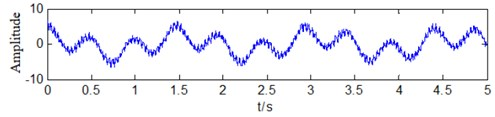Fig. 6. Mode signals extracted by IVMD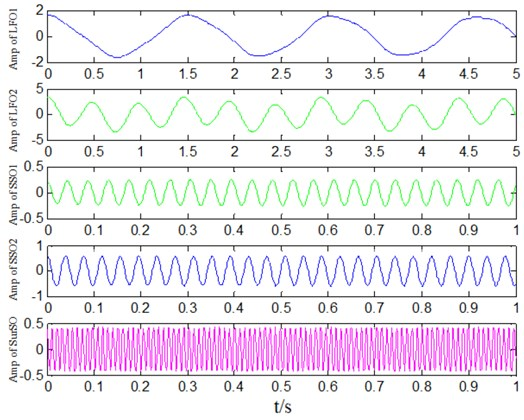The modal signals are extracted for each IVMD obtained in Fig. 6, and the modal identification is performed through the HTLS-Adaline algorithm to obtain the parameter information of the corresponding modal. The identification results of this method compared with those of other methods is shown in Table 2. The selected comparison methods are: (1) the classic EMD-based HTLS algorithm (EMD-HTLS); (2) the VMD-based HTLS algorithm (VMD-HTLS); (3) the proposed method is based on the algorithm of IVMD-HTLS and Adaline (IVMD-HTLS-Adaline).

Table 2. Mode identification of test signal

 Test signal EMD-HTLS VMD-HTLS Proposed method Modal Freq/ Hz Amp Phase/ rad Fre Amp Phase/ rand Freq / Hz Amp Phase/ rad Freq / Hz Amp Phase/ rad error ${y}_{LPO1}$ 0.65 2.35 1.0472 0.6648 2.2517 1.1815 0.6619 2.3841 0.9122 0.6512 2.3492 1.0488 2.029% 15.637% 12.82% 1.044% 5.199% 12.89% 0.264% 0.945% 0.153% ${y}_{LPO2}$ 2.05 3.00 0.6283 2.0641 3.1125 0.4868 2.4833 2.0855 0.7318 2.0525 3.0263 0.6338 4.321% 5.670% 22.52% 2.762% 4.775% 16.473% 0.233% 1.315% 0.875% ${y}_{SSO1}$ 25.56 0.58 0.5236 26.992 0.6233 0.6670 25.173 0.5922 0.5961 25.612 0.5842 0.5168 5.384% 23.122% 27.38% 2.231% 8.612% 13.846% 0.158% 4.122% 1.299% ${y}_{SSO2}$ 22.84 0.25 0.7854 25.237 0.2747 1.0141 22.157 0.2419 0.7741 22.774 0.2484 0.7880 10.13% 15.772% 29.11% 6.002% 5.409% 1.438% 0.487% 0.727% 0.331% ${y}_{SurSO}$ 92.32 0.42 1.0472 – – – 93.451 0.4438 1.1187 92.259 0.4291 1.0482 1.104% 7.437% 6.828% 0.215% 2.843% 0.096%

It can be seen from Table 2 that when the test signal oscillates in multiple frequency bands, the proposed method can effectively identify all oscillation modes. The maximum error of the oscillation frequency identification result is 1.55 %, with an average error of 0.67 %, and that of the oscillation amplitude identification result is 9.09 %, with an average error of 2.54 %. In comparison, the EMD-HTLS method has the worst frequency identification and amplitude identification results, and the average error is also the highest. The VMD-HTLS method has a good identification effect on the dominant oscillation mode (${y}_{LFO2}$) with the highest amplitude. The frequency and amplitude identification results are close to those of this method, but the phase identification results are not as good as those of this method. For the sub-modes ${y}_{LFO1}$ and ${y}_{SSO1}$, the VMD-HTLS frequency identification effect is close to the proposed method, but the amplitude and phase identification results are far worse than it. In particular, for mode ${y}_{SSO2}$ with low amplitude and high frequency, the recognition results of EEMD-HTLS and VMD-HTLS are poor. For the super-synchronous oscillation mode ${y}_{SurSO}$ with a frequency of 93.42, the EEMD-HTLS method failed to identify it, and the result of VMD-HTLS is inconsistent with the actual one. Therefore, the method proposed in this paper has obvious advantages in identifying multi-mode coexisting pan-band oscillations.

#### 4.2. Actual study data

In order to prove the effectiveness of the proposed method, the actual oscillation data of Hunan Power Grid was selected and the oscillation mode identification analysis was carried out. The oscillation event is as follows: On June 24, 2018, a low-frequency oscillation occurred in a thermal power plant in Hunan Power Grid. The system started to oscillate at low frequency in the 120th second. After 30 s, the system quickly started to emit an alarm. The system maintained an alarm for about 180 s. In 60-120 s before the oscillation, the system has a noise-like oscillation, and the system did not issue an alarm at this time. The range of the oscillation signal and noise-like signal has been marked in the figure. The system sampling frequency is 100 Hz. The oscillation signal at 165-195 s is selected, and the modal identification of this method is adopted. The original signal used for identification is shown in Fig. 7.

It can be seen from Fig. 6 that the amplitude of the oscillation signal is high, and the highest frequency fluctuation exceeds 100 MW, which is higher than 30 % of the system output power. At this time, the signal is affected by a certain degree of noise. For the original oscillation signal data shown in Fig. 7, the modal signals are extracted by the proposed algorithm as shown in Fig. 8. This method decomposes three modes from the oscillation signal data, which are the main modes of the system oscillation. Among the three modes, the first mode has the highest amplitude and is the dominant oscillation mode. The extracted modal signals are linearly superimposed, and the reconstructed signals are shown in Fig. 8.

Fig. 7. Original oscillation data used for mode identification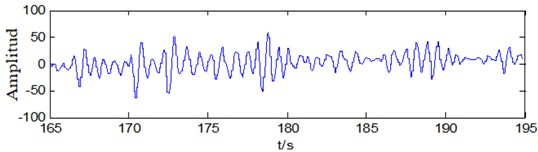Fig. 8. Mode signals extracted from the original oscillation data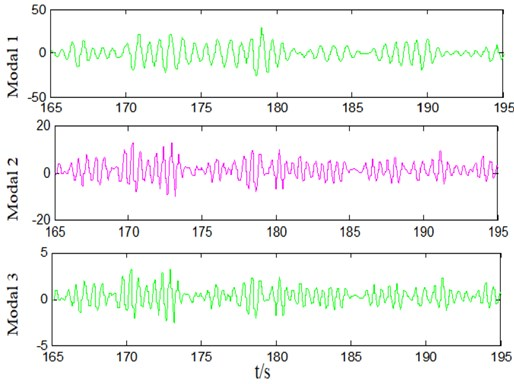Fig. 9. Reconstructed signal based on mode signals from IVMD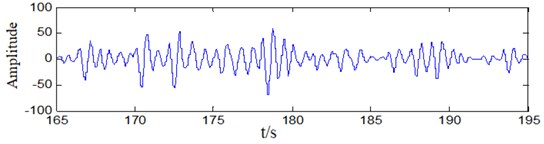Comparing Fig. 9 with Fig. 7, it is not difficult to find that the reconstructed signal is basically the same as the original signal waveform. That is, the three modal signals separated cover the main oscillations of the system. The method in this paper can effectively extract all the main oscillation modal signals. Using the Prony algorithm to perform parameter identification on the modal signals in Fig. 6, the information of the main oscillation modes can be obtained. Table 3 compares the results of this method with the MF-Prony and EMD-Prony parameter identification results. It can be seen from the results that for the oscillation signal, both the proposed method MF-Prony or EMD-Prony can identify the mode with the largest amplitude. In the three methods, the MF-Prony frequency identification error is the highest, and the results of this method are similar to the EMD-Prony method. During the severe oscillation, the dominant modal frequency is about 1.48 Hz, and the minor dominant modal frequency is about 2.02 Hz.

Table 3. Mode identification result and comparison of original oscillation data

 Method Modal Frequency / Hz Amplitude / MW Damping Phase / rad EEMD-HTLS 1 1.44 28.2 –1.18 2.6539 2 1.61 13.7 –1.59 0.2355 3 2.32 8.5 –2.31 2.9813 VMD-HTLS 1 1.48 30.6 –0.52 3.1348 2 1.51 16.3 –1.01 0.1653 3 1.08 8.7 –1.03 2.1981 IVMD-HTLS-Adaline 1 1.48 32.7 –0.94 3.0273 2 2.02 17.9 –1.40 0.0985 3 0.49 3.2 0.18 2.3499

#### 5. Conclusions

A VMD-based method for power system pan-band oscillation signal extraction and modal identification is proposed. This method can effectively extract low-frequency oscillation, sub-synchronous oscillation, and super-synchronous oscillation of multi-type and pan-band oscillations from power system operating data. Based on the oscillation discrimination, modal extraction and parameter identification, the identification results plays an important role in the analysis and control of system dynamic stability, as well as the identification and location of oscillation sources. It is helpful for early warning and timely suppression of system oscillation.

1. Kang Jie, Liu Li, Shao Yupei, Ma Qinggang Non-stationary signal decomposition approach for harmonic responses detection in operational modal analysis. Computers and Structures, Vol. 242, Issue 1, 2021, p. 106377. [Publisher]
2. David G. L., Jose R. R. H., Vicente V. H., Juan O. G., et al. Harmonic PMU and fuzzy logic for online detection of short-circuited turns in transformers. Electric Power Systems Research, Vol. 190, Issue 1, 2021, p. 106862. [Publisher]
3. Duan Guizhong, Qin Wenping, Lu Ruipeng, et al. Static voltage stability analysis considering the wind power and uncertainty of load. Power System Protection and Control, Vol. 46, Issue 12, 2018, p. 108-114. [Search CrossRef]
4. Zhang Xinran, Lu Chao, Liu Shichao, et al. A review on wide-area damping control to restrain inter-area low frequency oscillation for large-scale power systems with increasing renewable generation. Renewable and Sustainable Energy Reviews, Vol. 57, 2016, p. 45-58. [Publisher]
5. Li Shenghu, Sun Qi, ShiXuemei, et al. Suppression of weakly damped low-frequency modes of wind power system based on regional pole placement. Power System Protection and Control, Vol. 45, Issue 20, 2017, p. 14-20. [Search CrossRef]
6. Gao Haixiang, WuShuangxi, Miao Lu, et al. Overview of reasons for generator-induced power oscillations& its suppression measures. Smart Power, Vol. 46, Issue 7, 2018, p. 49-55. [Search CrossRef]
7. Suriyaarachchi D. H. R., Annakkage U. D., Karawita C., et al. A Procedure to study sub-synchronous interactions in wind integrated power systems. IEEE Transactions on Power Systems, Vol. 28, Issue 1, 2013, p. 377-384. [Publisher]
8. Karaagac U., Faried S. O., Mahseredjian J. Coordinated control of wind energy conversion systems for mitigating sub synchronous interaction in DFIG-based wind farms. IEEE Transactions on Smart Grid, Vol. 5, Issue 5, 2014, p. 2440-2449. [Publisher]
9. Cui Yahui, Zhang Junjie, Zhao Zongbin Sub synchronous oscillation torsional vibration model and simulation for a supercritical 600 MW unit with high voltage direct current transmission. Thermal Power Generation, Vol. 45, Issue 6, 2016, p. 106-110. [Search CrossRef]
10. Zhang Fan, Zhang Donghui, Liu Yongjun Risk assessment and suppression method study on sub-synchronous oscillation resulted from Luxi mixed back-to-back HVDC project. Smart Power, Vol. 45, Issue 4, 2017, p. 30-33. [Search CrossRef]
11. Xu Yanhui, Cao Yuping Research on mechanism of sub/sup-synchronous oscillation caused by GSC controller of direct-drive permanent magnetic synchronous generator. Power System Technology, Vol. 42, Issue 5, 2018, p. 1556-1564. [Search CrossRef]
12. Kun Liang, Xiaogong Lin, Yu Chen, Juan Li, Fuguang Ding Adaptive sliding mode output feedback control for dynamic positioning ships with input saturation. Ocean Engineering, Vol. 206, Issue 15, 2020, p. 107245. [Publisher]
13. Alejandro Garces Small-signal stability in island residential microgrids considering droop controls and multiple scenarios of generation. Electric Power Systems Research, Vol. 185, 2020, p. 106371. [Publisher]
14. Ayse Nihan Basmaci Characteristics of electromagnetic wave propagation in a segmented photonic waveguide. Journal of Optoelectronics and Advanced Materials, Vol. 22, Issues 9-10, 2020, p. 452-460. [Search CrossRef]
15. Ayse Nihan Basmaci Filiz Solution of Helmholtz equation using finite differences method in wires have different properties along X-axis. Journal of Electrical Engineering, Vol. 6, 2018, p. 206-211. [Publisher]
16. Zhineng Zhang, Ling Zheng 1D numerical study of nonlinear propagation of finite amplitude waves in traveling wave tubes with varying cross section. International Journal of Acoustics and Vibration, Vol. 25, Issue 1, 2020, p. 88-95. [Publisher]
17. Eldad J. A., Neeshtha D. B., Giuseppe C. G., Touvia M. Sound scattering by an elastic spherical shell and its cancellation using a multi-pole approach. Archives of Acoustics, Vol. 42, Issue 4, 2017, p. 697-705. [Publisher]
18. Mingsian Bai R., Jia Hong Lin, Kwan Liang Liu Optimized microphone deployment for near-field acoustic holography: to be, or not to be random, that is the question. Journal of Sound and Vibration, Vol. 329, Issue 14, 2010, p. 2809-2824. [Publisher]
19. Mraa Abad, Ahmadi H., Moosavian A., Khazaee M., Mohammadi M. Discrete wavelet transform and artificial neural network for gearbox fault detection based on acoustic signals. Journal of Vibroengineering, Vol. 15, Issue 1, 2013, p. 459-463. [Search CrossRef]
20. Wang Henan, Zheng Chao, Ren Jie Review on mechanism and analysis methods of low frequency oscillation in power system. Advanced Materials Research, Vol. 986, 2014, p. 2010-2013. [Publisher]
21. Wadduwage D. P., Annakkage U. D., Narendrak K. Identification of dominant low-frequency modes inring-down oscillations using multiple Prony models. IET Generation, Transmission and Distribution, Vol. 9, Issue 15, 2015, p. 2206-2214. [Publisher]
22. Jin Tao, Liu Dui Power grid low frequency oscillation recognition based on advanced Prony algorithm with improved denoising feature. Electric Machines and Control, Vol. 21, Issue 5, 2017, p. 33-41. [Search CrossRef]
23. Li Kuan, Li Xingyuan, Hu Nan ESPRIT analysis of sub synchronous oscillation based on the empirical mode decomposition self-adaptive filter. Power System Protection and Control, Vol. 40, Issue 13, 2012, p. 18-23. [Search CrossRef]
24. Zhang Yulin, Chen Hongwei Parameter identification of harmonics and inter-harmonics based on CEEMD-WPT and Prony algorithm. Power System Protection and Control, Vol. 46, Issue 12, 2018, p. 115-121. [Search CrossRef]
25. Wang Shen, Huang Songling, Wang Qing Mode identification of broadband Lamb wave signal with squeezed wavelet transform. Applied Acoustics, Vol. 125, 2017, p. 91-101. [Publisher]
26. Ren Zihui, Liu Haoyue, Xu Jinxia Power quality disturbance analysis based on wavelet transform and improved Prony method. Power System Protection and Control, Vol. 44, Issue 9, 2016, p. 122-128. [Search CrossRef]
27. Ye Y., Yuanzhang S., Lin C. Power system low frequency oscillation monitoring and analysis based on multi-signal online identification. Science China (Technological Sciences), Vol. 53, Issue 9, 2010, p. 2589-2596. [Publisher]
28. Jiang Ping, Shi Hao, Wu Xi Localizing disturbance source of power system forced oscillation caused by wind power fluctuation. Electric Power Engineering Technology, Vol. 37, Issue 5, 2018, p. 1-6. [Search CrossRef]
29. Xu Qi, Xu Jian, Shi Weil The forced oscillation analysis of wind integrated power systems based on singular value decomposition. Proceedings of the CSEE, Vol. 36, Issue 18, 2016, p. 4817-4827. [Search CrossRef]
30. Dragomiretskiy K., Zosso D. Variational mode decomposition. IEEE Transactions on Signal Processing, Vol. 62, Issue 3, 2014, p. 531-544. [Publisher]
31. Hauer J. F., Demeure C. J., Scharf L. L. Initial results in Prony analysis of power system response signals. IEEE Transactions on Power Systems, Vol. 5, Issue 1, 1990, p. 80-89. [Publisher]
32. Tang Guiji, Wang Xiaolong Parameter optimized variational mode decomposition method with application to incipient fault diagnosis of rolling bearing. Journal of Xi’an Jiaotong University, Vol. 49, Issue 5, 2015, p. 73-81. [Search CrossRef]
33. Ghodousian A., Parvari M. R. A modified PSO algorithm for linear optimization problem subject to the generalized fuzzy relational inequalities with fuzzy constraints. Information Sciences, Vol. 418, 2017, p. 317-345. [Publisher]
34. Liu Yanmin Research and Application of Particle Swarm Optimization. Ph.D. Dissertation, Shandong Normal University, China, 2011. [Search CrossRef]
35. Tang Guiji, Wang Xiaolong Application of variational modal decomposition method for optimizing parameters inearly fault diagnosis of rolling bearings. Journal of Xi’an Jiaotong University, Vol. 49, Issue 5, 2015, p. 73-81. [Search CrossRef]
36. Papy J. M., De Lathauwer L., Van Huffel S. Common pole estimation in multi-channel exponential data modeling. Signal Processing, Vol. 86, Issue 4, 2006, p. 846-858. [Publisher]